﻿ Dynamics
﻿

## Straight line motion

s: distance(m), t: time (s, second), v: velocity (m/s), VA: average velocity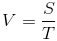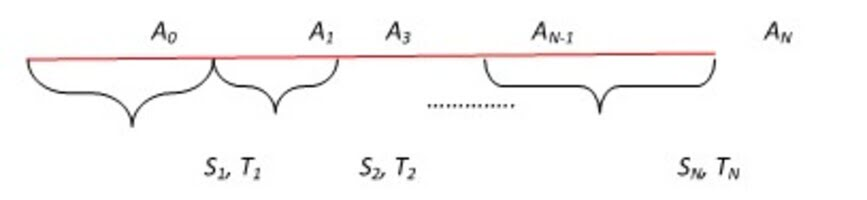## Constant Acceleration Straight Line Motion

A: Acceleration, V0: Initial Velocity, V: Velocity At Time T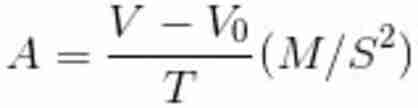## Distance Function

X0: Initial Distance From Origin, X: Distance At Time T## Uniform Circular Motion

Ø: angle (radian), l: length of an arc, R: radius of circle, ω: angular velocity (rad/s), t: time the object moves a length of l## Centripetal Force

f: frequency (Hz), T: the period for one rotation (s), a: centripetal acceleration, F: centripetal force (N, Newton)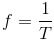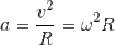## Constant Acceleration Circular Motion

γ: angular acceleration## Acceleration

at : tangential acceleration, an : normal acceleration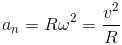## Freely Falling From Height H

g: gravitational acceleration, v: velocity at t, s: distance after t, vh: velocity when touching the ground, th: time to touch down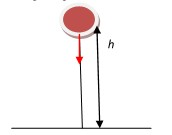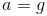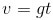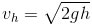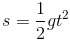g=9.8m/s2

## Vertical Projectile Motion

Hmax : maximum height, v0: initial velocity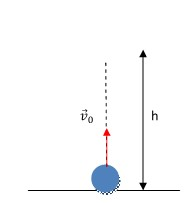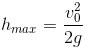Motion equation and velocity equation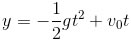## Angled Projectile Motion

vx, vy: velocity; x, y: motion equation by t; H: maximum height; L: maximum distance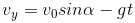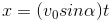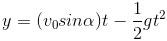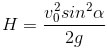## Horizontal Projectile Motion

th: time when touchdown, vh: velocity when touchdown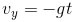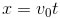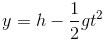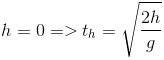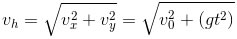## Sliding Motion On An Inclined Plane

µ: coefficient of some materials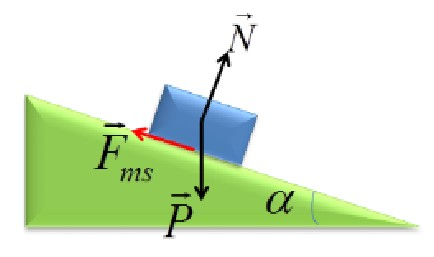Fiction coefficient of some materials

MaterialCoefficients of friction
Aluminium0.61
Rubber1.0
Wood0.62
Glass0.94